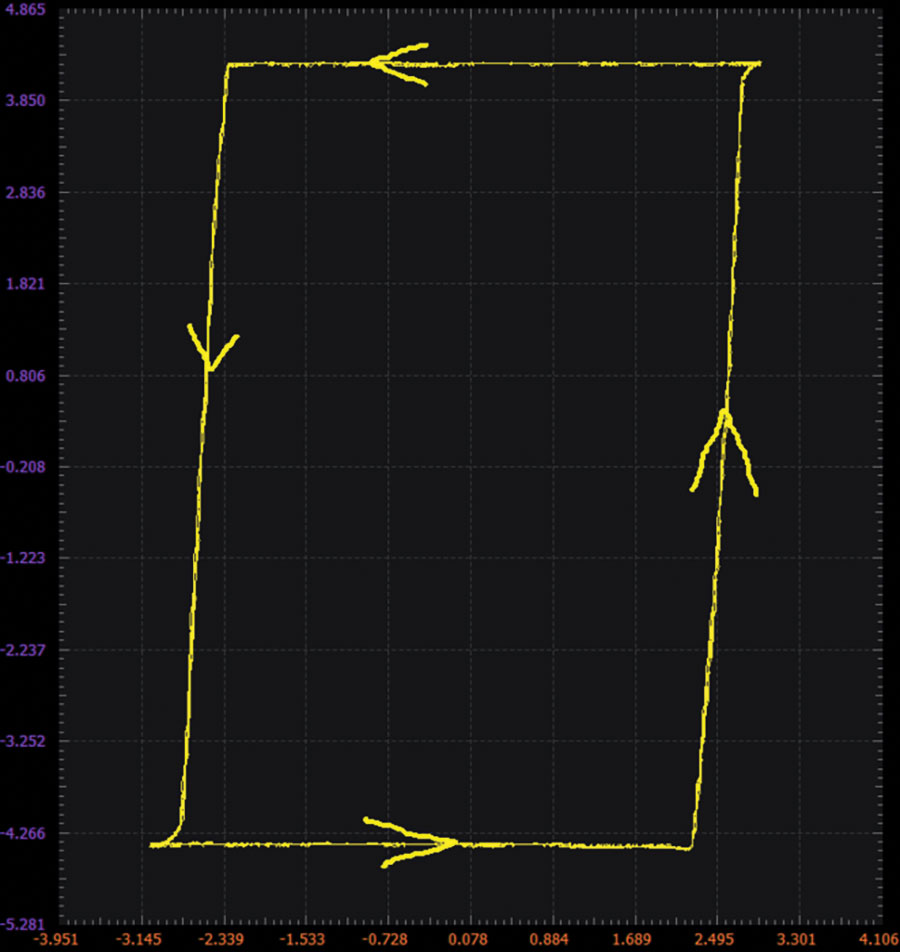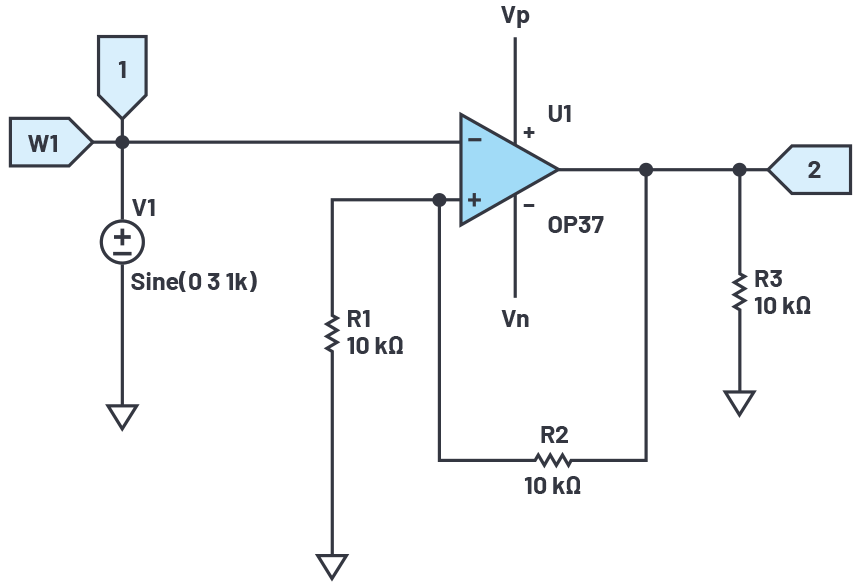# ADALM2000 Activity: Op Amp as Comparator

### Objective:

In this lab, we introduce the operational amplifier (op amp) in switch-mode configuration needed to obtain an op amp voltage comparator’s behavior. The voltage comparator circuit’s purpose is to highlight the relative state of the two-input voltage via two different states of the output voltage. The comparison is made using the sign of the difference between the two input voltages, while response is one of the two possible output values, dependent on the sign of that specific difference.

For an op amp comparator, we can consider a single input VD as the difference between V+ and V–. Therefore, the output voltage VO can get one of the two possible values:

• VO = VOH (high), meaning that V+ > V– (vD > 0)
• VO = VOL (low), meaning that V+ < V– (vD < 0)

We consider the threshold voltage VTh as the particular value (or values) of the input voltage vI for which the switching at the output takes place (setting VD = 0).

Two main types of voltage comparators are to be considered:

• Simple comparators: without feedback and with only one threshold voltage.
• Hysteresis comparators: with positive feedback and two threshold voltages.

#### Materials:

• ADALM2000 active learning module
• Solderless breadboard and jumper wire kit
• Three 10 kΩ resistor
• One 20 kΩ resistor
• One OP97 (low slew rate amplifier supplied with recent versions of the ADALP2000 analog parts kit) or OP37

### Simple Comparator

#### Background:

The high intrinsic gain of the op amp and the output satur ation effects can be exploited by configuring the op amp as a comparator as in Figure 1. This is essentially a binary-state decision-making circuit: if the voltage at the positive (+) terminal is greater than the voltage at the negative (–) terminal, VIN > VREF, the output goes high (saturates at its maximum value). Conversely, if VIN < VREF the output goes low. The circuit compares the voltages at the two inputs and generates an output based on the relative values. Unlike all the previous circuits, there is no feedback between the input and output; we say that the circuit is operating open-loop.Figure 1. Op amp as comparator.

#### Hardware Setup:

Comparators are used in different ways, and we will see them in action in future labs. Here we will use the comparator in a common configuration that generates a square wave with a variable pulse width.

Start by shutting off the power supplies and assemble the circuit. As with the summing amplifier circuit, use the second waveform generator output for the dc source VREF, and turn the amplitude to zero and the output offset all the way down so that you can adjust up from zero during the experiment.

Configure the waveform generator VIN for a 2 V amplitude peak-to-peak sine wave at 1 kHz. With the po wer supply on and VREF at zero volts, export the output waveform.

Now slowly increase VREF and observe what happens. Record the output waveform for VREF = 1 V. Keep increasing VREF until it exceeds 2 V and observe what happens. Can you explain this?

Repeat the previous step for a triangular input waveform and record your observations for your lab report.

#### Procedure:

Use the first waveform generator as source VIN to provide a 2 V amplitude peak-to-peak, 1 kHz sine wave excitation to the circuit. Supply the op amp to ±5 V from the power supply. Configure the scope so that the input signal is displayed on Channel 1 and the output signal is displayed on Channel 2.

A plot example is presented in Figure 3.

### Hysteresis Comparator

Hysteresis is the dependence of a system’s current state on previous values of quantities determining said current state. The output value is not a strict function of the corresponding input, but also incorporates some lag, delay, or history dependence. In particular, the response for a decrease in the input variable is different from the response for an increase in the input variable.

In this configuration, there are two threshold values VThH and VThL with two output values VOH and VOL. The threshold values should depend on the output value, which is fed back to the input and contributes to the threshold values (positive feedback). Via a resistive divider, a fraction of the output voltage is fed back to the noninverting input.

When analyzing hysteresis comparators, we must take into consideration the moving direction of the hysteresis and the fact that, at a certain moment, only one threshold is active.

The input signal triggers the switching of the output, with the switching process being sustained by the positive feedback.

### Noninverting Hysteresis Comparator

#### Background:

Consider the circuit presented in Figure 4.Figure 4. Noninverting hysteresis comparator.

For the noninverting hysteresis comparator circuit in Figure 4, VIN is applied to the noninverting input of the op amp. Resistors R1 and R2 form a voltage divider network across the comparator, providing the positive feedback with part of the output voltage appearing at the noninverting input along with the VIN via the same resistive divider.

The amount of feedback is determined by the resistive ratio of the two resistors used (in this situation, the ratio will be one-half).

We can compute the threshold voltages as follows:

Considering VD = 0, VIN → VTh, we obtain the following thresholds:

#### Hardware Setup:

Build the following breadboard circuit for the noninverting hysteresis comparator.

#### Procedure:

Use the first waveform generator as source VIN to provide a 6 V amplitude peak-to-peak, 1 kHz sine wave excitation to the circuit. Supply the op amp to ±5 V from the power supply. Configure the scope so that the input signal is displayed on Channel 1 and the output signal is displayed on Channel 2.

A plot example is presented in Figure 6.Figure 6. Noninverting hysteresis comparator waveform.

In Figure 7, you can observe the voltage transfer characteristic of the noninverting hysteresis comparator (the arrows drawn indicate the flow of the signal with respect to the thresholds). Compare the computed threshold voltage values with the measured ones.Figure 7. Noninverting hysteresis comparator XY plot.

### Inverting Hysteresis Comparator

#### Background:

Consider the circuit presented in Figure 8.Figure 8. Inverting hysteresis comparator.

For the inverting hysteresis comparator circuit in Figure 8, VIN is applied to the inverting input of the op amp. Resistors R1 and R2 form a voltage divider network across the comparator providing the positive feedback with part of the output voltage appearing at the noninverting input.

The amount of feedback is determined by the resistive ratio of the two resistors used (in this situation, the ratio will be one-half).

We can compute the threshold voltages as follows:

Considering VD = 0, VIN → VTh, we obtain the following thresholds:

#### Hardware Setup:

Build the following breadboard circuit for the inverting hysteresis comparator.

#### Procedure:

Use the first waveform generator as source VIN to provide a 6 V amplitude peak-to-peak, 1 kHz sine wave excitation to the circuit. Supply the op amp to ±5 V from the power supply. Configure the scope so that the input signal is displayed on Channel 1 and the output signal is displayed on Channel 2.

A plot example is presented in Figure 10.Figure 10. Inverting hysteresis comparator waveform.

In Figure 11, you can observe the voltage transfer characteristic of the noninverting hysteresis comparator (the arrows drawn indicate the flow of the signal with respect to the thresholds). Compare the computed threshold voltage values with the measured ones.Figure 11. Inverting hysteresis comparator XY plot.

### Inverting Hysteresis Comparator with Asymmetric Thresholds

#### Background:

Consider the circuit presented in Figure 12.Figure 12. Inverting hysteresis comparator with asymmetric thresholds.

For the inverting comparator with asymmetric thresholds circuit in Figure 12, an additional reference voltage VREF is used. Resistors R1 and R2 form a voltage divider network across the comparator providing the positive feedback with part of the output voltage appearing at the noninverting input, along with a part of the VREF going through the same divider.

We can compute the threshold voltages as follows:

Considering VD = 0, VIN → VTh, we obtain the following thresholds:

#### Hardware Setup:

Build the following breadboard circuit for the inverting hysteresis comparator.Figure 13. Inverting hysteresis comparator with asymmetric thresholds breadboard.

#### Procedure:

Use the first waveform generator as source VIN to provide a 6 V amplitude peak-to-peak, 1 kHz sine wave excitation to the circuit and second waveform generator as a constant 2 V voltage reference. Supply the op amp to ±5 V from the power supply. Configure the scope so that the input signal is displayed on Channel 1 and the output signal is displayed on Channel 2.

A plot example is presented in Figure 14.Figure 14. Inverting hysteresis comparator with asymmetric thresholds waveform.

In Figure 15, you can observe the voltage transfer characteristic of the noninverting hysteresis comparator (the arrows drawn indicate the flow of the signal with respect to the thresholds). Compare the computed threshold voltage values with the measured ones.Figure 15. Inverting hysteresis comparator with asymmetric thresholds XY plot.

### Question:

Compute the threshold voltages for all four comparator setups (simple, noninverting hysteresis, inverting hysteresis, and asymmetric thresholds) and compare the results with the ones obtained from the experimental setups.

### Extra Activities

For experimenters who finish early or want an additional challenge, see if you can modify the comparator circuit using your red and green LEDs (from the last lab) at the output so that the red LED lights for negative voltages and the green LED lights for positive voltages. Turn down the frequency to 1 Hz (or less) so you can see them turn on-and-off in real time. Don’t forget that the LEDs will need a current-limiting resistor so that the current through it is no more than 20 mA.

You can also extend the above example to a circuit with multiple voltage levels as the circuit presented in Figure 16.Figure 16. Voltage level indicator using LEDs.

#### Materials:

• ADALM2000 active learning module
• Solderless breadboard and jumper wire kit
• Three 470 Ω resistor
• One 10 kΩ resistor
• Two 20 kΩ resistor
• Three LEDs (red, green, yellow)
• One ADTL082 (two integrated op amps)

The circuit uses a divider (R1, R2, R3) to obtain one threshold for each of the two comparators. Based on these thresholds and the input voltage, one LED (D1, D2, D3) at a time will be on.

### Exercises:

• Compute the threshold voltages according to the circuit in Figure 16. Determine which LED will be on for each input voltage range.
• Build the breadboard circuit. Supply the op amp to ±5 V from the power supply. Use the first channel of the signal generator to generate the variable input voltage (VIN) and the second channel to generate the 5 V constant reference voltage.Figure 17. Voltage level indicator using LEDs.

Vary the input voltage from 0 V to 5 V and observe the LEDs’ behavior.

This type of circuit is also known as a window comparator. An application on this subject can be found in the activity: Temperature Control Using Window Comparator.

You can find more details at the StudentZone blog.